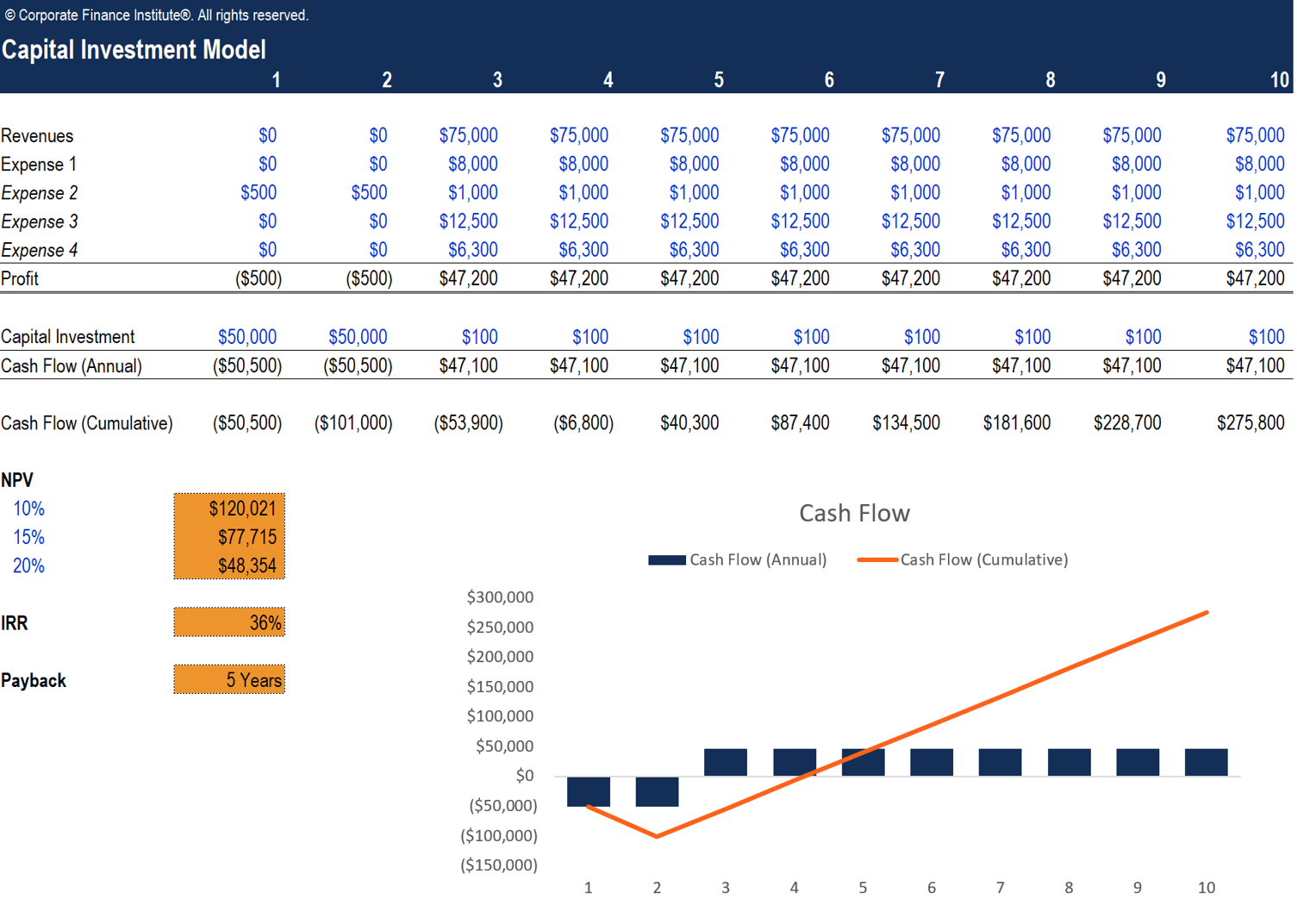# Capital Investment Model

Evaluate the return and payback on a company's capital investment

## What is a Capital Investment Model

Most companies make long-term investments which require a large amount of capital invested in the initial years, mostly in fixed assets such as property, machinery, or equipment. Due to the significant amount of cash outflows required, companies would always perform a capital investment analysis to evaluate the profitability of the investment and determine whether it is worthy. This is especially important when a business is presented with multiple potential opportunities and need to make a investment decision based on the long-run returns they can get.

To assess the profitability of a capital investment, companies can build a capital investment model in Excel to calculate key valuation metrics including the cash flows, net present value (NPV), internal rate of return (IRR), and payback period.

In this guide, we will outline the major line items that should be included in a capital investment model and how to use the calculated metrics to evaluate the investment.### #1 Revenues, Expenses and Profit

The first step to building a capital investment model is to determine the cash flows for the investment period. In this simplified model, we are presenting the income statement using the minimal number of line items – revenue, expenses, and profit. By subtracting the expenses from the annual revenue we can determine the profit for each year within the investment period, which will be used as cash inflows for the capital investment.

### #2 Capital Investment and Cash Flow

Next, we need to determine the amount of capital invested into the project which equals to the the cash outflows during the investment period. With that information, we can then calculate the annual cash flows using the following formula:

Cash Flow (Annual) = Profit – Capital Investment

The cash flow line will be the major input in the calculations of net present value (NPV) and internal rate of return (IRR) for this capital investment.

We also need to determine the cumulative cash flow, which is essentially the sum of all cash flows from the beginning of investment period to present year. The cumulative cash flow figures will be used to compute the payback period of the investment.

### #3 Net Present Value (NPV)

The net present value (NPV) is the value of all future cash flows over the entire life of the capital investment discounted to the present. In this example, we will determine the NPV using three different discount rates – 10%, 15%, and 20%. This rate is often a company’s weighted average cost of capital (WACC), or the required rate of return investors expect to earn relative to the risk of the investment.

In Excel, you can use the NPV function to find the present value of a series of future cash flows with equal time periods. The formula for the NPV function is: = NPV(rate, cash flows).

In this example, the NPV of this capital investment would be \$120,021 when the discount rate is 10%, \$77,715 when discount rate is 15%, and \$48,354 when discount rate is 20%. This tells us that when there is higher risk associated with a capital investment, investors expect to pay less today and expects a higher return in the future.

### #4 Internal Rate of Return (IRR)

The internal rate of return (IRR) is the expected compound annual rate of return earned on a capital investment. IRR is usually compared to a company’s WACC to determine whether an investment is worthy or not.  If the IRR is greater than or equal to the WACC, the company would accept the project as a good investment. Vice versa, the company would reject the investment if the IRR is less than the WACC.

You can use the IRR function in Excel to compute the rate of return based on a series of future cash flows. The formula for the IRR function is: =IRR(rate, cash flows).

### #5 Payback Period

The last metric to calculate for a capital investment is the payback period, which is the total time it takes for a business to recoup its investment. Payback period is similar to a breakeven analysis but instead of the number of units to cover fixed costs, it looks at the amount of time required to return the investment.

A simple way to calculate the payback period is to count the number of periods until the company earns a positive cumulative cash flow on the investment. In the example provided, the company starts to have positive cumulative cash flow in year 5 and thus the payback period for this investment is 5 years.

### #6 Cash Flow Chart

Beside calculating the payback period, a cash flow chart is also a good way to visualize the cash flow trend over the investment period and the time when the investment breaks even. In the example above, the column chart in blue represents the annual cash flow of the investment while the line chart in orange shows the cumulative cash flow over the period. The point where the orange line intersects with the horizontal axis is the breakeven point, where the company earns zero cumulative cash flow and begins to recognize positive cash flow after paying back all initial investment.